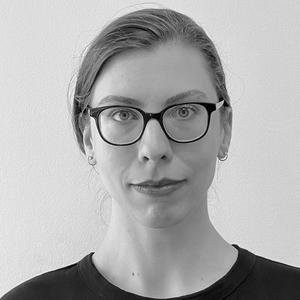## Tracey Balehowsky, BSc (Hon.), MSc, PhD### Areas of Research

Geometric inverse problems
In such problems I often look for what geometric information on (pseudo-) Riemannian manifolds one can use to determine the metric. See papers https://arxiv.org/abs/2011.09312v2 and https://arxiv.org/abs/1711.09379 for example of my work on such problems.
Transformation optics and cloaking
In these problems one is looking to mathematically model the construction a device (called a cloaking device) which distorts optical waves to render an object invisible to an observer. In particular, I have looked at a use case for such a device for modelling the optical properties of manifolds which represent a geometric configuration for our universe. See https://arxiv.org/abs/2011.09547v2 for an example of such a problem.
Geometric flows
Geometric flows are PDEs which describe how a shape (a (pseudo-) Riemannian manifold) can be deformed according to some aspect of the geometric. Such flows include mean curvature flow (MCF) and Ricci flow. I am particularly interested in studying MCF and Ricci flow as they appear in related geometric or physical questions. See https://arxiv.org/abs/1110.0765 and https://arxiv.org/abs/1004.1833 for examples.
Particle kinematics
Particle kinematics can be modelled by a Boltzmann equation, which describes particle motion and accounts for interactions between particles, such as particle collisions and particle evaporation. I have studied such an equation from a theoretical inverse problem perspective (see https://arxiv.org/abs/2011.09312v2) and from an applied atmospheric science perspective (see https://acp.copernicus.org/articles/20/15867/2020/acp-20-15867-2020-discussion.html). I would be happy to supervise a thesis related to these research avenues.

### Supervising degrees

Math and Statistics - Masters: Seeking Students
Math and Statistics - Doctoral: Seeking Students